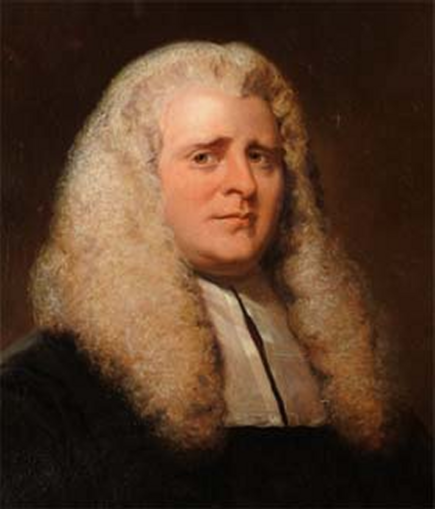# Wilson’s TheoremJohn Wilson (Mathematician)See page for author [Public domain], via Wikimedia CommonsWilson’s Theorem is a test for primality.  It is inefficient for large numbers since factorials ($$n!$$) grow very fast.  Nevertheless, it has its uses because of its simplicity.

It was conjectured by Wilson and published in a book by Waring in 1770.  It was proved by Lagrange in 1773.  A manuscript of 1682 indicates that Leibniz was aware of the result.

If and only if $$p$$ is a prime number, then

$$\displaystyle \frac {(p-1)! + 1}{p}$$

is a natural number.

In modular arithmetic, we write Wilson’s Theorem as the congruence

$$\bbox[10px,border:2px solid black]{(p-1)!\equiv -1\pmod p}$$

The converse of Wilson’s Theorem is also true.

If $$n \gt 1$$ is a natural number and $$(n-1)!\equiv -1\pmod n$$, then $$n$$ is prime.

For a composite number, $$n$$, $$(n-1)!\equiv 0\pmod n$$ except when $$n = 4$$.

For small values we have

$$\begin{array}{r|r|r|r} n & (n-1)! & (n-1)!\pmod n & Prime?\\ \hline 2 & 1 & 1 & T\\ 3 & 2 & 2 & T\\ 4 & 6 & 2 & F\\ 5 & 24 & 4 & T\\ 6 & 120 & 0 & F\\ 7 & 720 & 6 & T\\ 8 & 5040 & 0 & F\\ 9 & 40320 & 0 & F\\ 10 & 362880 & 0 & F\\ 11 & 3628800 & 10 & T \end{array}$$

For example :

$$7$$ is prime.

$$(7-1)! = 6! = 1 \times 2 \times 3 \times 4 \times 5 \times 6 = 720$$

Using

$$\displaystyle \frac {(p-1)! +1}{p} = n$$

We have

$$\displaystyle \frac {6! + 1}{7} = \frac {721}{7} = 103$$

In modular arithmetic, we write this as the congruence

$$6! \equiv -1 \pmod 7$$

One way to explain how this works is by using modular multiplicative inverses.

Ordinary multiplication gives :

$$\begin {array}{c|cccccc} \times & 1 & 2 & 3 & 4 & 5 & 6\\ \hline 1 & 1 & 2 & 3 & 4 & 5 & 6\\ 2 & 2 & 4 & 6 & 8 & 10 & 12\\ 3 & 3 & 6 & 9 & 12 & 15 & 18\\ 4 & 4 & 8 & 12 & 16 & 20 & 24\\ 5 & 5 & 10 & 15 & 20 & 25 & 30\\ 6 & 6 & 12 & 18 & 24 & 30 & 36 \end{array}$$

Multiplication in modulo $$7$$ gives the residues (remainders) :

$$\begin {array}{c|cccccc} \times & 1 & 2 & 3 & 4 & 5 & 6\\ \hline 1 & 1 & 2 & 3 & 4 & 5 & 6\\ 2 & 2 & 4 & 6 & 1 & 3 & 5\\ 3 & 3 & 6 & 2 & 5 & 1 & 4\\ 4 & 4 & 1 & 5 & 2 & 6 & 3\\ 5 & 5 & 3 & 1 & 6 & 4 & 2\\ 6 & 6 & 5 & 4 & 3 & 2 & 1 \end{array}$$

By inspection of the above table, we see that $$2$$ and $$4$$ and $$3$$ and $$5$$ are modular multiplicative inverses of each other since $$1$$ is the multiplicative identity. $$1$$ and $$6$$ are both self-inverse.

Thus, we can write

$$6! \equiv 1 \times 1 \times 1 \times 1 \times 1 \times 6 \pmod 7 \equiv 1 \times 6 \pmod 7 \equiv -1 \pmod 7$$

© OldTrout $$2019$$